# Test: Heron's Formula- 1

## 25 Questions MCQ Test Mathematics (Maths) Class 9 | Test: Heron's Formula- 1

Description
This mock test of Test: Heron's Formula- 1 for Class 9 helps you for every Class 9 entrance exam. This contains 25 Multiple Choice Questions for Class 9 Test: Heron's Formula- 1 (mcq) to study with solutions a complete question bank. The solved questions answers in this Test: Heron's Formula- 1 quiz give you a good mix of easy questions and tough questions. Class 9 students definitely take this Test: Heron's Formula- 1 exercise for a better result in the exam. You can find other Test: Heron's Formula- 1 extra questions, long questions & short questions for Class 9 on EduRev as well by searching above.
QUESTION: 1

Solution:
QUESTION: 2

### The sides of a triangle are in the ratio of 3 : 4 : 5. If its perimeter is 36 cm, then what is its area?

Solution:

It is given that the sides of a triangle are in the ratio 3 : 4 : 5.

Let the length of sides are 3x, 4x and 5x.

It's perimeter is 36 cm.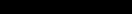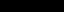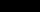The value of x is 3. The length of sides are 9, 12, 15.

It is an right angled triangle because the sum of squares of two smaller sides is equal to the square of larger sides.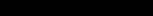Here, the length of hypotenuse is 15 cm.

The area of triangle is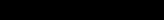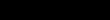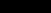QUESTION: 3

### An isosceles right triangle has area 8 cm2. The length of its hypotenuse is

Solution:

area of a triangle = base x height/2

for a right angled isosceles triangle, base = height

so area = base2/2

8 x2 = base2

base = height = 4 cm

hypotnuese = √base2 + height2 = √2base2

= √2 x 8 x 2

= √32 cm

QUESTION: 4

The cost of turfing a triangular field at the rate of Rs. 45 per 100 m2 is Rs. 900. If the double the base of the triangle is 5 times its height, then its height is

Solution:

Let the height of triangular field be h metres.

It is given that 2 x (base) = 5 × (Height)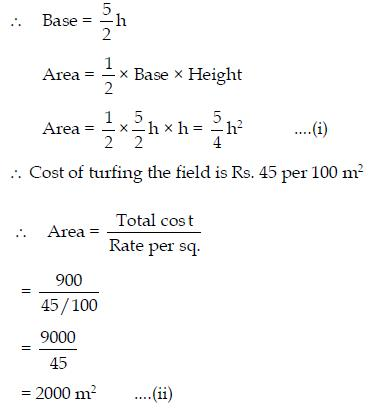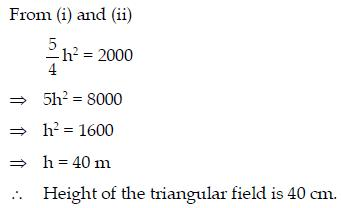QUESTION: 5

The area of the the triangle having sides 1 m, 2 m and 2 m is :

Solution:
Through Heron’s formula =
S=Perimeter/2
S=5/2
Let a=1m, b=2m ,c=2m

Formulae= Root(s(s-a)(s-b)(s-c))
=root(5/2(5/2 - 1)(5/2 - 2)(5/2 - 2))
=root(5/2*3/2*1/2*1/2)
=root(15/16)
=root(15)/4
QUESTION: 6

The base of a right triangle is 8 cm and hypotenuse is 10 cm. Its area will be

Solution:
QUESTION: 7

The area of a triangle whose sides are 12 cm, 16 cm and 20 cm is

Solution:

Solution :- Area of a triangle of sides a , b & c is : √( s(s-a)(s-b)(s-c) )

where s=(a+b+c)/2

ln given triangle , s=(20+12+16)/2=48/2=24

Thus area of given triangle = √(24(24-20)(24-12)(24-16))

=√(24×4×12×8)

=√9216

=96

Thus area of triangle of sides 20cm ,12cm and 16cm is 96cm2

QUESTION: 8

The difference of semi-perimeter and the sides of △ABC are 8, 7 and 5 cm respectively. Its semi-perimeter ‘s’ is

Solution: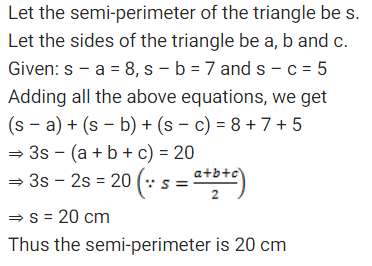QUESTION: 9

The sides of a triangle are 56 cm, 60 cm and 52 cm long. Then the area of the triangle is

Solution:
QUESTION: 10

The sides of a triangular flower bed are 5 m, 8 m and 11 m. the area of the flower bed is

Solution:
QUESTION: 11

A triangle ABC in which AB = AC = 4 cm and ∠A = 90o, has an area of

Solution:
QUESTION: 12

The perimeter and area of a triangle whose sides are of lengths 3 cm, 4 cm and 5 cm respectively are

Solution:
QUESTION: 13

The perimeter of a rhombus is 20 cm. One of its diagonals is 8 cm. Then area of the rhombus is

Solution:

Given, Perimeter of a rhombus = 20 cm
Perimeter of a rhombus = 4*side
Hence, side = 20/4 = 5 cm.
Now, we know that the diagonals of a rhombus bisect each other at right angles (90 degree).
Hence 'a right angled triangle can be visualised with 'side' as the hypotenuse'.

diagonal length = 8 cm
Half the length (since diagonal bisects each other) = 8/2 = 4 cm
(d/2)2 + (d1/2)^2 = 52
4^2 + (d1/2)2 = 52
(d1/2)2 = 9
d1/2 = 3
d1 = 3*2 = 6 cm
Hence other diagonal = 6 cm.

Area = 1/2 * d1*d = 1/2 * 8 * 6 = 24 cm2

QUESTION: 14

The area of an isosceles triangle having base 2 cm and the length of one of the equal sides 4 cm, is

Solution: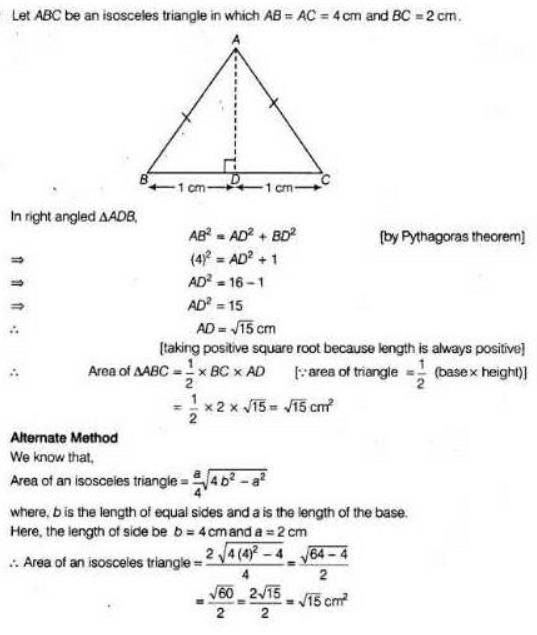QUESTION: 15

The length of the sides of a triangle are 5 cm, 7 cm and 8 cm. Area of the triangle is :

Solution:
QUESTION: 16

If the side of an equilateral triangle is 4 cm, then its area is

Solution:
QUESTION: 17

The area of a triangle whose sides are 15 cm, 8 cm and 19 cm is

Solution:
QUESTION: 18

If one side and one diagonal of a rhombus and 20 m and 24 m, then its area =

Solution:

The rhombus has a perimeter of 80m.
A rhombus has 4 equal sides, each would be 20m.
One of the diagonals is 24m...this is bisected by the other diagonal into 12m segs.
The diagonals of a rhombus intersect at right angles. Use the Pythagorean Theorem to find the length of the other diagonal...
12² + b² = 20²
144 + b² = 400
b² = 256
b = 16
This is of course the length of just one segment, the diagonal is 32m.
Now we can use our area formula with the diagonals.
A = ½d¹d²
A = ½(24)(32)
A = 384 m²

QUESTION: 19

The edges of a triangular board are 6 cm, 8 cm and 10 cm. The cost of painting it at the rate of 9 paise per cmis

Solution:
QUESTION: 20

Area of an equilateral triangle of side 2 cm is :

Solution:
QUESTION: 21

If the perimeter of an equilateral triangle is 24 m, then its area is

Solution:

Given:
Area of equilateral ∆ = 16√3 m²
Let the side of equilateral ∆ = a m
Area of equilateral ∆ = (√3/4)a²
16√3 = (√3/4)a²
16√3 × (4/√3) = a²
64 = a²
a = √64
a =√ 8 × 8 = 8 m
Side of equilateral ∆ = 8 m
Perimeter of equilateral ∆ = 3 × side
Perimeter of equilateral ∆ = 3 × 8 = 24 m.
Hence, the perimeter of equilateral ∆ is 24 m.

QUESTION: 22

Each of the equal sides of an isosceles triangle is 2 cm greater than its height. If the base of the triangle is 12 cm, then its area is

Solution: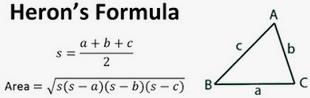and, equating this with the basic area formula,

i.e. A = 1/2.h.b

QUESTION: 23

The product of difference of semi-perimeter and respective sides of △ABC are given as 13200 m3. The area of △ABC, if its semi-perimeter is 132m, is given by

Solution:
QUESTION: 24

The area of equilateral triangle of side ‘a’ is 4√3 cm2. Its height is given by

Solution:
QUESTION: 25

The base of a right triangle is 8 cm and hypotenuse is 10 cm. Its area will be :

Solution:
Given,

base= 8cm

hypotenuse= 10cm

First, let us find the height of the triangle:

Let height = h

By applying pythagorean theorem

h 2 + 8 2 = 10 2

h 2 + 64 = 100

h 2 = 100 - 64

h 2 = 36

h = 6cm

Now let us find the area,

Area = 1/2 x b x h

= 1/2 x 8 x 6

= 1/2 x 48

= 24cm 2

Therefore, area of the right triangle = 24cm 2